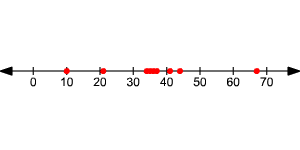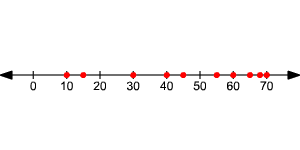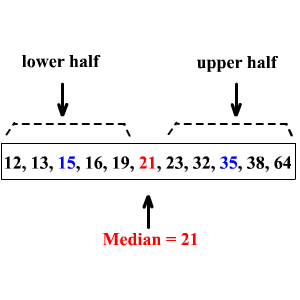# Variation of Data

One simple way to measure the variation of a data set is its range.

Example :

Consider the set of values: .

The highest value of the data set is $\text{67}$ and the lowest is $\text{10}$ . So, the range of the data set is

$67-10=57$

But that doesn't tell the whole story. Sometimes, we are also interested in how clustered or spread out the data is.

Consider another set of data .

The two sets have almost the same range, but the distributions have different shapes.

If you draw a line plot of the two, it will look like this:In the first data set, the data is clustered around the median, $36.5$ .

In the second data set, the data is more spread out, with a little cluster near the top of the range.

In a set of data, the quartiles are the values that divide the data into four equal parts. The median of a set of data separates the set in half.The median of the lower half of a set of data is the lower quartile (LQ) or ${Q}_{1}$ .

The median of the upper half of a set of data is the upper quartile (UQ) or ${Q}_{3}$ .

Here, ${Q}_{1}=15$ and ${Q}_{3}=35$

The upper and lower quartiles can be used to find another measure of variation call the interquartile range.

The interquartile range is the range of the middle half of a set of data. It is the difference between the upper quartile and the lower quartile.

Interquartile range = ${Q}_{3}-{Q}_{1}$

In the above example, the interquartile range is $35-15=20$ .

Data points that are more than $1.5$ times the value of the interquartile range beyond the quartiles are called outliers.